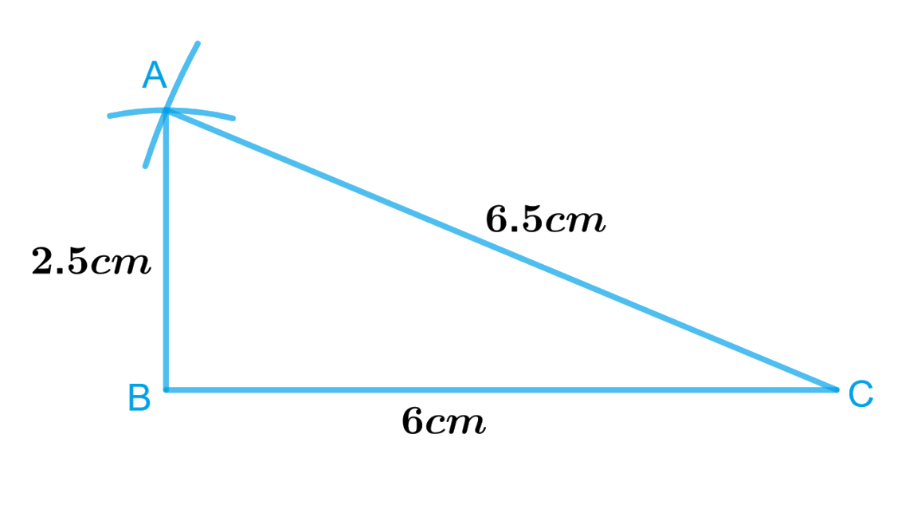# Ex.10.2 Q4 Practical Geometry Solution - NCERT Maths Class 7

## Question

Construct $$ΔABC$$ such that $$AB = 2.5\,\rm{cm}$$., $$BC = 6\,\rm{cm}$$. and $$AC = 6.5\,\rm{cm}$$.. Measure $$∠B.$$

Video Solution
Practical Geometry
Ex 10.2 | Question 4

## Text Solution

What is known?

Lengths of sides of a triangle are $$AB = 2.5 \,\rm{cm}$$., $$BC = 6\,\rm{ cm}$$. and $$AC = 6.5\,\rm{ cm}$$..

To construct:

A $$ΔABC$$ such that $$AB = 2.5\,\rm{cm}$$., $$BC = 6\,\rm{cm}$$. and $$AC = 6.5\,\rm{cm}$$.. Measure $$∠B.$$

Reasoning:

To Construct $$ΔABC$$ such that $$AB = 2.5 \,\rm{cm}, \,BC = 6 \,\rm{cm}$$ and $$AC = 6.5\,\rm{ cm}$$. and to measure $$∠B$$, follow the steps given below.

Steps:Steps of construction:

1. Draw a line segment $$BC$$ of length $$6\,\rm{ cm}$$..
2. From $$B$$, point $$A$$ is at a distance of $$2.5\,\rm{ cm}$$.. So, with $$B$$ as centre, draw an arc of radius $$2.5\,\rm{ cm}$$. (now $$A$$ will be somewhere on this arc & our job is to find where exactly $$A$$ is).
3. From $$C$$, point $$A$$ is at a distance of $$6.5\,\rm{ cm}$$.. So, with $$C$$ as centre, draw an arc of radius $$6.5\,\rm{ cm}$$. (now $$A$$ will be somewhere on this arc, we have to fix it).
4. $$A$$ has to be on both the arcs drawn, so it is the point of intersection of arcs. Mark the point of intersection of arcs as $$A$$ join $$AB$$ and $$AC.$$

Thus, $$ABC$$ is the required triangle.

Measure angle $$B$$ with the help of protractor. It is the right-angled triangle $$ABC$$, where $$∠B =$$ $$90^\circ.$$

Learn from the best math teachers and top your exams

• Live one on one classroom and doubt clearing
• Practice worksheets in and after class for conceptual clarity
• Personalized curriculum to keep up with school